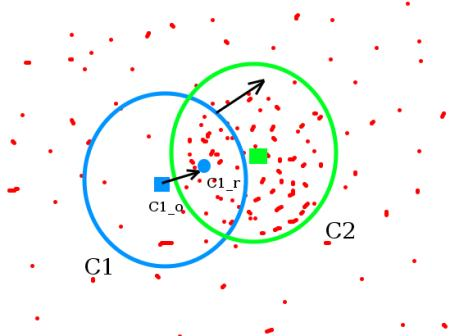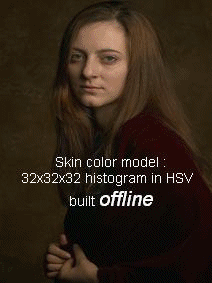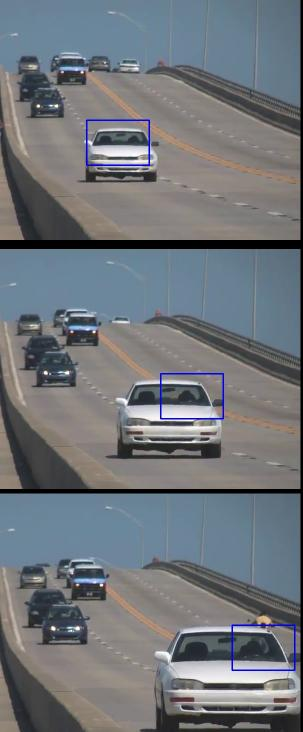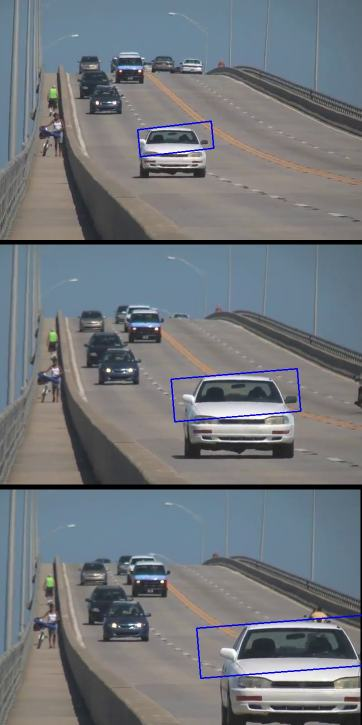# Meanshift 和 Camshift

## 目标

• 我们将学习如何让利用 Meanshift 和 Camshift 算法用以在视频中寻找并跟踪对象

Meanshift 中译均值漂移聚类， Camshift 则是连续自适应 Meanshift 算法，

## Meanshift

Meanshift 背后的直观感受是很简单的。想象一下，你有一堆的随机散列点(这些点可以是像直方图反向投影那样的像素分布)。同时你拥有一个小窗口(或许是一个圆圈)，现在你需要移动这个窗口直到窗口内的像素密度最大(或者说所含点的数量最多)。这在下面这幅图像中是很容易说明的：meanshift imagemeanshift face image

### 在 opencv 中使用 Meanshift

import numpy as np
import cv2 as cv

cap = cv.VideoCapture('slow.flv')

# 获取视频的第一帧

# 设置窗口的初始位置
r,h,c,w = 250,90,400,125  # 简单地硬编码值
track_window = (c,r,w,h)

# 设置 ROI(图像范围)以进行跟踪
roi = frame[r:r+h, c:c+w]
hsv_roi =  cv.cvtColor(roi, cv.COLOR_BGR2HSV)
mask = cv.inRange(hsv_roi, np.array((0., 60.,32.)), np.array((180.,255.,255.)))
cv.normalize(roi_hist,roi_hist,0,255,cv.NORM_MINMAX)

# 设置结束标志，10 次迭代或至少 1 次移动
term_crit = ( cv.TERM_CRITERIA_EPS | cv.TERM_CRITERIA_COUNT, 10, 1 )

while(1):

if ret == True:
hsv = cv.cvtColor(frame, cv.COLOR_BGR2HSV)
dst = cv.calcBackProject([hsv],,roi_hist,[0,180],1)

# 运行 meanshift 算法用以获取新的位置
ret, track_window = cv.meanShift(dst, track_window, term_crit)

# 绘制到新图像中
x,y,w,h = track_window
img2 = cv.rectangle(frame, (x,y), (x+w,y+h), 255,2)
cv.imshow('img2',img2)

k = cv.waitKey(60) & 0xff
if k == 27:
break
else:
cv.imwrite(chr(k)+".jpg",img2)
else:
break
cv.destroyAllWindows()
cap.release()meanshift result image

## Camshiftcamshift face image

### 在 OpenCV 里使用 Camshift

import numpy as np
import cv2 as cv

cap = cv.VideoCapture('slow.flv')

# 获取视频的第一帧

# 设置窗口的初始位置
r,h,c,w = 250,90,400,125  # 简单地硬编码值
track_window = (c,r,w,h)

# 设置 ROI(图像范围)以进行跟踪
roi = frame[r:r+h, c:c+w]
hsv_roi =  cv.cvtColor(roi, cv.COLOR_BGR2HSV)
mask = cv.inRange(hsv_roi, np.array((0., 60.,32.)), np.array((180.,255.,255.)))
cv.normalize(roi_hist,roi_hist,0,255,cv.NORM_MINMAX)

# 设置结束标志，10 次迭代或至少 1 次移动
term_crit = ( cv.TERM_CRITERIA_EPS | cv.TERM_CRITERIA_COUNT, 10, 1 )

while(1):

if ret == True:
hsv = cv.cvtColor(frame, cv.COLOR_BGR2HSV)
dst = cv.calcBackProject([hsv],,roi_hist,[0,180],1)

# 运行 Camshift 用以获取新的位置
ret, track_window = cv.CamShift(dst, track_window, term_crit)

# 绘制到新图像中
pts = cv.boxPoints(ret)
pts = np.int0(pts)
img2 = cv.polylines(frame,[pts],True, 255,2)
cv.imshow('img2',img2)

k = cv.waitKey(60) & 0xff
if k == 27:
break
else:
cv.imwrite(chr(k)+".jpg",img2)
else:
break
cv.destroyAllWindows()
cap.release()camshift result image

## 其他资源

1. 关于Camshift的法语维基百科页面。(上面的两幅动图出自于此)
2. Bradski, G.R.的 “Real time face and object tracking as a component of a perceptual user interface “论文

## 练习

1. OpenCV 附带了一个关于 camshift 交互式演示的 Python 示例。使用它，拆解它，理解它。